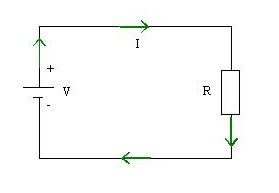# Electrical resistance and Ohm's law

Electrical resistance (R) is the friction or obstacles that are given a substance to an electrical current. With this friction or obstacles, causing the motion of electrons decreases. Barriers that hinder electron movement is called the resistance, while the instrument is called a resistor or resistance. Unit to measure electrical resistance is called Ohm or by the symbol Ω (read Omega).

The greater the resistance of a conductor, the smaller the electrical current flowing to him.

Ohm's law
The relationship between electric current, voltage, and electrical resistance in a circuit expressed in Ohm's lawWhere:
- V shows the line voltage to the units of volts (V)
- I shows the electrical current to the units of Ampere (A)
- R indicates a unit of electrical resistance Ohm (Ω)

Sample questions:
See the picture below. Given the value of electric current (I) for 6 Ampere, and the value of resistance (R) of 15 Ω. What is the required voltage (V)?Completion:
V = I x R = 6 x 15 = 90 Volts

Next→
←Prev